#Bernoulli EquationsA differential equation of Bernoulli type is written as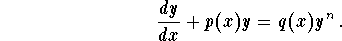This type of equation is solved via a substitution. Indeed, let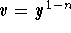. Then easy calculations give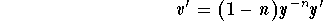which implies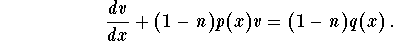This is a linear equation satisfied by the new variable v. Once it is solved, you will obtain the function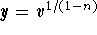. Note that if n > 1, then we have to add the solution y=0 to the solutions found via the technique described above.
Let us summarize the steps to follow:

(1)
Recognize that the differential equation is a Bernoulli equation. Then find the parameter n from the equation;
(2)
Write out the substitution;
(3)
Through easy differentiation, find the new equation satisfied by the new variable v.
You may want to remember the form of the new equation:(4)
Solve the new linear equation to find v;
(5)
Go back to the old function y through the substitution;
(6)
If n > 1, add the solution y=0 to the ones you obtained in (4).
(7)
If you have an IVP, use the initial condition to find the particular solution.

Example: Find all the solutions for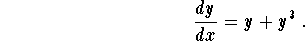Solution: Perform the following steps:

(1)
We have a Bernoulli equation with n=3;
(2)
Consider the new function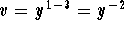;
(3)
The new equation satisfied by v is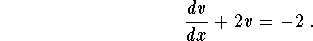;

(4)
This is a linear equation:
4.1
the integrating factor is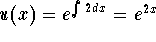4.2
we have4.3
the general solution is given by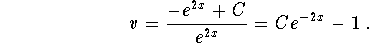5
Back to the function y: we have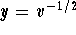, which gives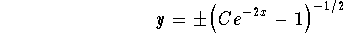6
All solutions are of the form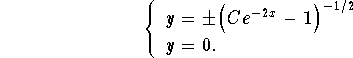[Differential Equations]
[Algebra] [Trigonometry ]
[Calculus] [Complex Variables] [Matrix Algebra]S.O.S. MATHematics home page

Do you need more help? Please post your question on our S.O.S. Mathematics CyberBoard.Author: Mohamed Amine Khamsi
Last Update 6-23-98